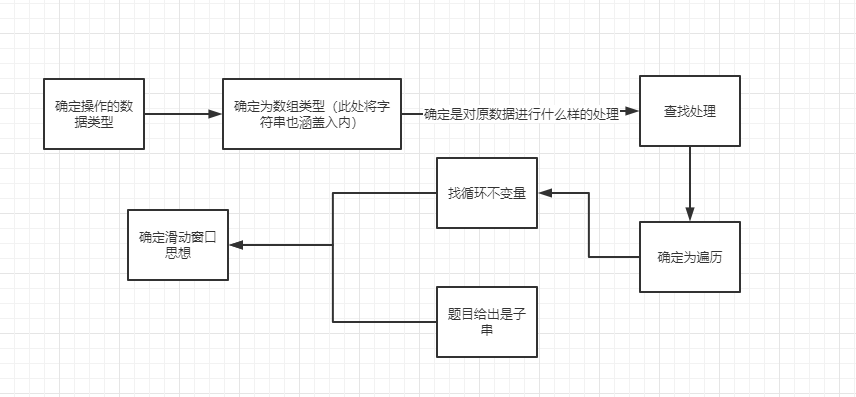`中等`

``````示例 1:

请注意，你的答案必须是 子串 的长度，"pwke" 是一个子序列，不是子串。

### 题目分析

`滑动窗口`属于`双指针`思想的一种，所谓`双指针`并不是我们编程语言中指内存地址的那个指针，而是一种技巧，通过两个变量去动态地对我们访问（或者说遍历）的数据进行标记（不明白的继续往下看，等下会有演示）。

`滑动窗口`思想之所以如此取名，其实就是双指针在遍历的时候，会形象地成为一个数据域的两个端点，而数据域中的数据往往是我们所需要的数据，就像是一个窗口一样(想象成窗户，通过窗户我们能看到风景，而窗户旁边则是墙，墙后面的风景我们是看不到的，也是不关心的)。在遍历的过程中，两个指针会根据判别不断地移动，像是在数组上不断滑动的窗口。

``````我们先假设给出的字符串是："abcabcbb"

↓
abcabcbb
↑

1、right向右移动，发现 [left, right] 这个数据域中没有重复元素，right 继续移动

↓
abcabcbb
↑

2、假设此时遍历到第二个 a, 如果此时 right 移动到第二个 a 上的话，[left, right]这个数据域中便会出现不满足条件的数据，即发现有重复子串。
↓
abcabcbb
↑### 题解 - 滑动窗口 + Map

``````/**
* @param {string} s
* @return {number}
*/
var lengthOfLongestSubstring = function(s) {
const len = s.length

if (len < 2) return len

const cut = new Map()

let left = 0,
right = 0
let ans = 1

while (right < len) {
// 记录元素出现次数
cut.set(s[right], (cut.get(s[right])|| 0) + 1)
if (cut.get(s[right]) === 2) {
// 判断元素重复
while (cut.get(s[right]) === 2) {
cut.set(s[left], cut.get(s[left ++]) - 1)
}
}
// 每次更新最长子串的长度
ans = Math.max(ans, right - left + 1)
right ++
}

return ans
};``````

### 题解 - 滑动窗口 + Map 进一步优化，减少 left 移动次数

``````/**
* @param {string} s
* @return {number}
*/
var lengthOfLongestSubstring = function(s) {
const len = s.length

// 特判
if (len < 2) return len

const map = new Map()

let left = 0,
right = 0,
ans = 1

while (right < len) {

const point = map.get(s[right])

// 如果 point 为 undefined 的话，说明先前并未出现过此元素，所以没有重复
if (point !== undefined) {
left = Math.max(left, point + 1)
}
// 更新元素出现的位置
map.set(s[right], right)
ans = Math.max(ans, right - left + 1)
right ++
}

return ans
};``````